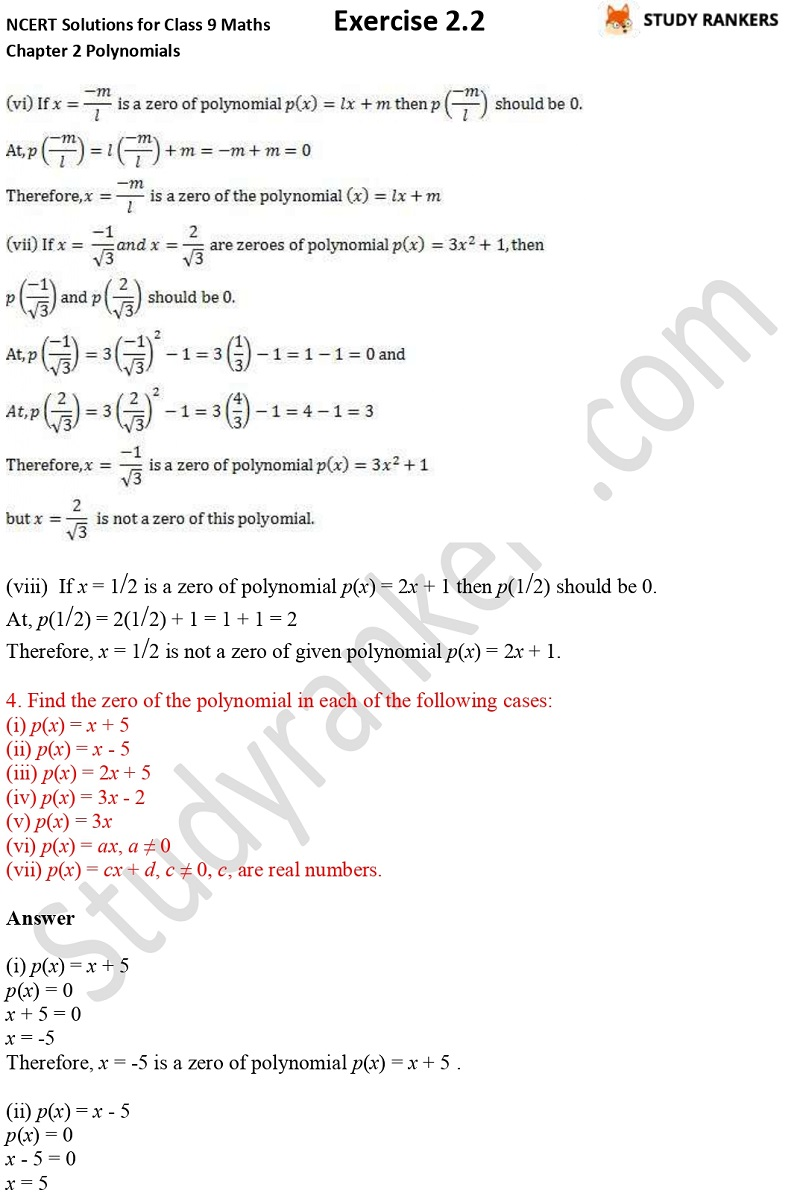## NCERT Solutions for Class 9 Maths Chapter 2 Polynomials Exercise 2.2

Chapter 2 Polynomials Exercise 2.2 Class 9 Maths NCERT Solutions provided here that will help you in completing homework. You can take help from this page if you ever face problem in finding accurate NCERT Solutions for Class 9 Maths so it will build your basics. By practicing the questions and answers, you will develop your problem solving skills that will be useful in solving difficult questions in the exams.

Exercise 2.2 has four questions that are about finding the value and zeroes of given polynomials.